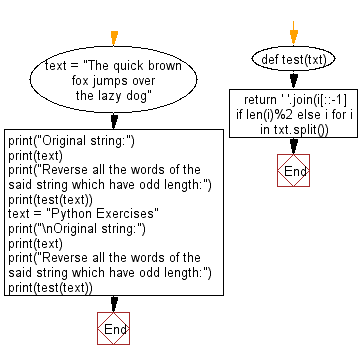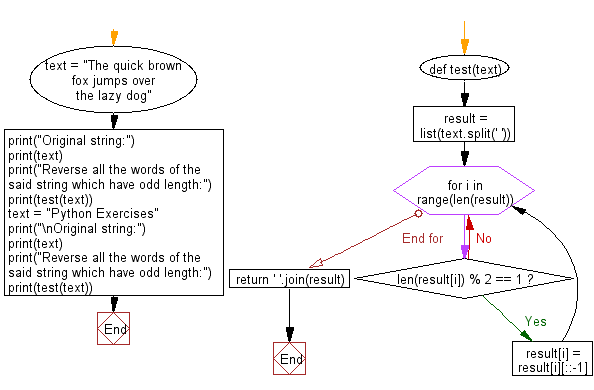﻿ Python: Reverse all the words which have odd length - w3resource

# Python: Reverse all the words which have odd length

## Python Basic - 1: Exercise-136 with Solution

Write a Python program to reverse all words of odd lengths.

Sample Solution-1:

Python Code:

``````def test(txt):
return ' '.join(i[::-1] if len(i)%2 else i for i in txt.split())

text = "The quick brown fox jumps over the lazy dog"
print("Original string:")
print(text)
print("Reverse all the words of the said string which have odd length:")
print(test(text))
text = "Python Exercises"
print("\nOriginal string:")
print(text)
print("Reverse all the words of the said string which have odd length:")
print(test(text))
``````

Sample Output:

```Original string:
The quick brown fox jumps over the lazy dog
Reverse all the words of the said string which have odd length:
ehT kciuq nworb xof spmuj over eht lazy god

Original string:
Python Exercises
Reverse all the words of the said string which have odd length:
Python sesicrexE
```

Flowchart:Sample Solution-2:

Python Code:

``````def test(text):
result = list(text.split(' '))
for i in range(len(result)):
if len(result[i]) % 2 == 1:
result[i] = result[i][::-1]
return ' '.join(result)

text = "The quick brown fox jumps over the lazy dog"
print("Original string:")
print(text)
print("Reverse all the words of the said string which have odd length:")
print(test(text))
text = "Python Exercises"
print("\nOriginal string:")
print(text)
print("Reverse all the words of the said string which have odd length:")
print(test(text))
``````

Sample Output:

```Original string:
The quick brown fox jumps over the lazy dog
Reverse all the words of the said string which have odd length:
ehT kciuq nworb xof spmuj over eht lazy god

Original string:
Python Exercises
Reverse all the words of the said string which have odd length:
Python sesicrexE
```

Flowchart:## Visualize Python code execution:

The following tool visualize what the computer is doing step-by-step as it executes the said program:

Python Code Editor:

Have another way to solve this solution? Contribute your code (and comments) through Disqus.

What is the difficulty level of this exercise?

Test your Programming skills with w3resource's quiz.

﻿